# AP 7th Maths Mock Test 2023 For Chapter – 5 : ” Triangles And Its Properties ” Online Practice Test

1. It two angles in a triangle are 750 , 550 what type of triangle is that ?
2. The ration in which the centroid of a triangle divides the median is
3. From the adjacent figure find the values of x and y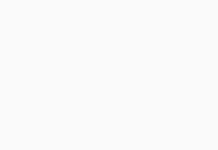4. The angles of the triangle are in the ratio 1 : 2 : 3 then the smallest angle is
5. A triangle can have .................. altitudes
6. The exterior angle of a triangle is 1300 and one of its interior opposite angles is 600 , then the other opposite interior angle is
7. If in a triangle two angles are equal and the third angle is 1200 , what are the equal angles ?
8. Find x and y values from the figure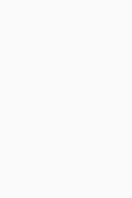9. An obtuse angled triangle has ..................... acute angles
10. The angle in an equilateral triangle is
11. In a right angled isosceles triangle the acute angle is
12. An acute angled triangle has ..................... acute angles
13. A triangle which has maximum two acute angles is
14. The following are acute angled triangles
15. The following is the possible third side if the two sides are 6 cm , 9 cm
16. Match the following
1. AB + BC >                             ( ) a . BC
2. BC - CA <                              ( ) b. CA
17. The altitude of the ................ triangle lies outside of the triangle
18. What is x here ?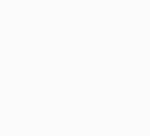19. In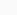ABC if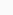A = 3B andC = 2B find all the three angles ofABC
20. In the adjacent figure x0 =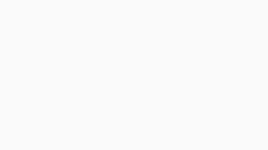21. Which of the following are the possible sides of a triangle ?
22. If the three angles of a triangle are in the ratio 1 : 2 : 3 then the angles are
23. InXYZ ,X = 300 ,Y = 450 then findZ =
24. In the given figure , the values of x + y is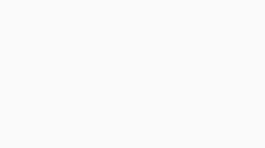25. Angles of a triangle are 300 , 1100 , x0then x is
26. The lengths of two sides of an isosceles triangle are 7 cm , 8 cm then the possible third side is of length
27. The exterior angle of an equilateral triangle is
28. If the angles are in the ratio 3 : 1 : 2 then biggest angle is
29. The two angles of a triangle are complementary then it is .......................... triangle
30. Find 'x' in the figure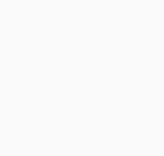31. Find 'z' in the figure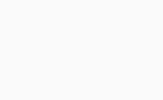32. In aPQR , ifP = 1000 andQ =R thenP +R =
33. Match the following
1. 600 , 600 , 600           ( ) a. Obtuse angled triangle
2. 450 , 450 , 450              ( ) b. Isosceles triangle
3. 1000 , 400 , 400         ( ) c. Right angled triangle
4. 900 , 300 , 600            ( ) d. Equilateral triangle
5. 500 , 500 , 800           ( ) e. Right angled isosceles triangle
34. Least number of possible acute angles in a triangle is.........
35. Which type of triangle is formed by BC = 7.2 cm , AC = 6 cm andC = 1200 ?
36. Which triangle is formed by AB = 3 cm , BC = 4 cm and AC = 8 cm ?
37. P : An isosceles triangle is right angled
Q :A =B = 450 andC = 900Which of the following statement is true ?
38. Which of the following statement is not true ?
39. Which of the following angles are not the angles of a triangle ?
40. Sum of interior angles in a triangle is equal to
41. Some of two acute angles of right angled triangle is
42. InABC , which of the following is false ?
43. A triangle can have ............... obtuse angle
44. The relation between x and y in the given figure expressed with 'y' as subject is ............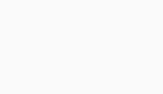45. Following lengths of the sides of a triangle are given . In which case it is not possible to construct a triangle (in cm)
46. The sum of interior angles in a pentagon is ..........
47. The opposite interior angles are in the ratio 1 : 4 thenA ,B ?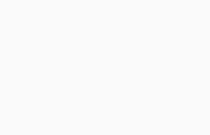48. In the adjacent figureA +B +C +D +E = ...........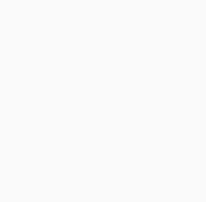49. Find the value of x and y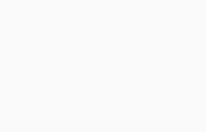50. The following is the representation of line segment
51. One of the exterior angle of a triangle is 1050 and the interior opposite angles are in the ratio 2 : 5 Find the angles of the triangle
52. If an exterior angle of a triangle is 1300 and one of the interior opposite angle is 600 then find the other interior opposite angle
53. The exteriorPRS ofPQR is 1050 . IfQ = 700 findP then relation betweenPRS andP is
54. One of the exterior angles of a triangle is 1250 and the interior opposite angles are in the ratio 2 : 3 then find the angles of triangle
55. The acute angles of a right - triangle are in the ratio 2 : 3 then find the angles of the triangle
56. If the angles of a triangle are in the ratio 1 : 4 : 5 then angles are
57. InPQR ,P = 2Q and 2R = 3Q calculate the angles ofPQR
58. The angles of triangle are in the ratio 1 : 2 : 3 then greatest angle is
59. The angles of triangle are in the ratio 1 : 2 : 3 then angles are
60. Which of the following is true about triangle ?
61. In a right angled triangle , one acute angle is 300 then find other acute angle
62. Find the measure of the third angle of triangles whose two angles are 380 , 1020
63. Find the measure of the third angle of triangles whose two angles are 1160 , 300
64. Find the measure of the third angle of triangles whose two angles are 400 , 800
65. In a right angled triangle , one acute angle is 600 then find other acute angle
66. The angles of triangle are in the ratio 2 : 3 : 4 then angles are
67. The acute angles of a right - triangle are in the ratio 1 : 2 then find the angles of the triangle
68. If the angles of a triangle are in the ratio 2 : 3 : 4 then angles are
69.  InXYZ ,X = 2Y andZ = 3Y calculate the angles ofXYZ
70.  InDEF ,D = 4E and 2F = 10E calculate the angles ofDEF
71. If an exterior angle of a triangle is 1200 and one of the interior opposite angle is 700 then find the other interior opposite angle
72. If an exterior angle of a triangle is 1400 and one of the interior opposite angle is 400 then find the other interior opposite angle
73. One of the exterior angle of a triangle is 1000 and the interior opposite angles are in the ratio 2 : 3 Find the angles of the triangle
74. One of the exterior angle of a triangle is 1600 and the interior opposite angles are in the ratio 1 : 3 Find the angles of the triangle
75. Which of the following are impossible ?
76. Triangle has ................ line segments
77. Triangle is
78. Triangle has .............. vertices
79. Triangle has .............. sides
80. Triangle has .............. angles
81. HereA is opposite to side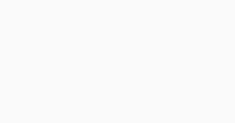82. HereC is opposite to side83. Here opposite angle to side CA is84. Here opposite angle to side BA is85. Triangle formed by 3 collinear points with equal distance formed ....................... triangle
86. The region of plane enclosed byABC is called the
87. The region of plane is not enclosed byABC is called the
88. The interior of a triangle together with its boundary is known as the ............................. in a plane
89. A triangle having all the three sides are equal length is called
90. A triangle having at least two sides of equal length is called
91. A triangle having all three sides with different lengths is called
92. A triangle whose angles are less than 900 is called
93. A triangle whose angles are greater than 900 is called
94. A triangle whose angles is 900 is called
95. In Angle - sum property of a triangle the sum of all three angles in triangle is
96. A line segment drawn form a vertex of triangle which is perpendicular to the side opposite to the vertex is called an
97. The line segment that joins a vertex and the mid point of its opposite side is called
98. The point of intersection of median of a triangle is called
99. Centroid of triangle is denoted by
100. In triangle means